Courses

# Fourier Series - Mathematical Methods of Physics, UGC - NET Physics Physics Notes | EduRev

## Physics for IIT JAM, UGC - NET, CSIR NET

Created by: Akhilesh Thakur

## Physics : Fourier Series - Mathematical Methods of Physics, UGC - NET Physics Physics Notes | EduRev

The document Fourier Series - Mathematical Methods of Physics, UGC - NET Physics Physics Notes | EduRev is a part of the Physics Course Physics for IIT JAM, UGC - NET, CSIR NET.
All you need of Physics at this link: Physics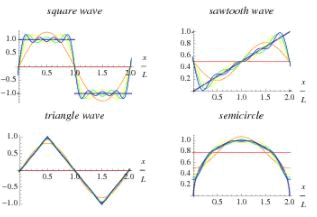A Fourier series is an expansion of a periodic function f(x) in terms of an infinite sum of sines and cosines. Fourier series make use of the orthogonality relationships of the sine and cosine functions. The computation and study of Fourier series is known as harmonic analysis and is extremely useful as a way to break up an arbitrary periodic function into a set of simple terms that can be plugged in, solved individually, and then recombined to obtain the solution to the original problem or an approximation to it to whatever accuracy is desired or practical. Examples of successive approximations to common functions using Fourier series are illustrated above.

In particular, since the superposition principle holds for solutions of a linear homogeneous ordinary differential equation, if such an equation can be solved in the case of a single sinusoid, the solution for an arbitrary function is immediately available by expressing the original function as a Fourier series and then plugging in the solution for each sinusoidal component. In some special cases where the Fourier series can be summed in closed form, this technique can even yield analytic solutions.

Any set of functions that form a complete orthogonal system have a corresponding generalized Fourier series analogous to the Fourier series. For example, using orthogonality of the roots of a Bessel function of the first kind gives a so-called Fourier-Bessel series.

The computation of the (usual) Fourier series is based on the integral identities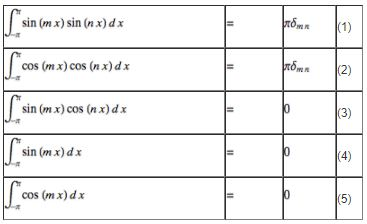for m,n ≠ o where  δmn is the Kronecker delta.

Using the method for a generalized Fourier series, the usual Fourier series involving sines and cosines is obtained by taking  f1 (x) = cos x and  f2 (x)  = sin x. Since these functions form a complete orthogonal system over [- π, π ] the Fourier series of a function  f (x) is given by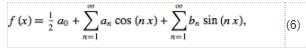where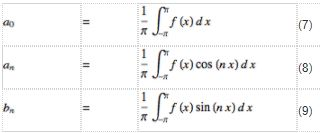and n = 1, 2, 3, .... Note that the coefficient of the constant term a0 has been written in a special form compared to the general form for a generalized Fourier series in order to preserve symmetry with the definitions of an and bn.

A Fourier series converges to the function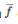(equal to the original function at points of continuity or to the average of the two limits at points of discontinuity)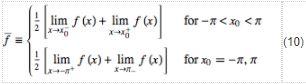if the function satisfies so-called Dirichlet boundary conditions. Dini's test gives a condition for the convergence of Fourier series.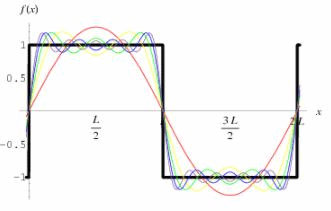As a result, near points of discontinuity, a "ringing" known as the Gibbs phenomenon, can occur.

For a function f(x) periodic on an interval [-L, L] instead of  [- π, π ], a simple change of variables can be used to transform the interval of integration from  [- π, π ] to [-L, L]. Let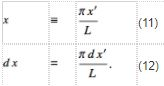Solving for x' gives x' = Lx/πx'  and plugging this in gives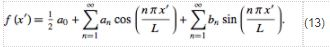Therefore,Similarly, the function is instead defined on the interval [0,2L], the above equations simply become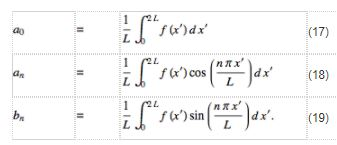In fact, for f(x) periodic with period 2L, any interval  (x0, x0 + 2L) can be used, with the choice being one of convenience or personal preference .

The coefficients for Fourier series expansions of a few common functions are given in Beyer and Byerly. One of the most common functions usually analyzed by this technique is the square wave. The Fourier series for a few common functions are summarized in the table below.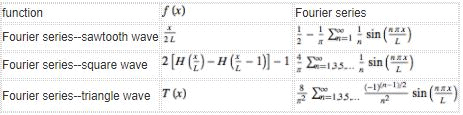If a function is even so that f(x) = f (-x) , then f(x) sin (n, x) is odd. (This follows since sin (n, x) is odd and an even function times an odd function is an odd function.) Therefore, bn = 0 for all n. Similarly, if a function is odd so that f(x) = -f (-x), then f(x) cos (n, x)  is odd. (This follows since cos (n, x) is even and an even function times an odd function is an odd function.) Therefore, an = 0 for all n.

The notion of a Fourier series can also be extended to complex coefficients. Consider a real-valued function  f(x) . Write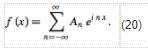Now examine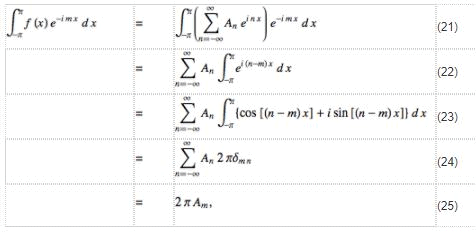so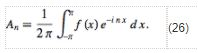The coefficients can be expressed in terms of those in the Fourier series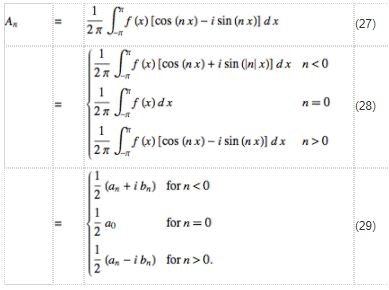For a function periodic in [-L/2, L/2], these become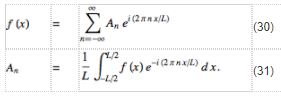These equations are the basis for the extremely important Fourier transform, which is obtained by transforming An from a discrete variable to a continuous one as the length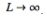.

159 docs

,

,

,

,

,

,

,

,

,

,

,

,

,

,

,

,

,

,

,

,

,

,

,

,

;